# How to Round Numbers in Google Sheets? (ROUNDUP/ROUNDDOWN)

The rounding function in Google sheets will convert the decimal number to the nearest whole number, the second decimal point, hundreds, and by the factor of three. Google Sheets makes it easy to round the numbers with various rounding functions like ROUND, ROUNDUP, ROUNDDOWN, MROUND, and so on. In this article, let’s learn everything about Round Function with the help of Google Sheet tips provided on this page.

## ROUND Function in Google Sheets

The ROUND function can reduce or increase any value by a specific number of decimal places. The rounding digit will be rounded up or rounded down.

### Rounding Number to the Nearest Integer Number in Google Sheets

To round any decimal value to the nearest numeric value(integer) we can use the ROUND function in Google sheets.

• If the number to the right of the rounding digit is less than five, the rounding digit is not changed.
• If the number to the right of the rounding digit has a value of five or higher, the rounding digit is raised by one.

Below is the syntax for the ROUND function in google sheets,

=ROUND(value)

value – we should specify the decimal number which has to be round.

Example,

• =ROUND(2.6) – This will give you 3
• =ROUND(2.4) – This will give you 2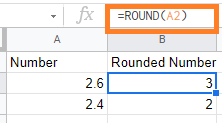In the above example,

• The decimal value 2.6 is converted to integer value 3. Since the right side number is greater than 5 the google sheets ROUND function is rounding up.
• The decimal value 2.4 is converted to integer value 2. Since the right side number is lesser than 5 the google sheets ROUND function is rounding down.

The round formula in google sheets will round up or round down the decimal value to the nearest integer value.

### Rounding Numbers to Nearest Decimal Point in Google Sheets

To round any decimal value to the nearest decimal point, we can use the ROUND function in Google sheets.

Below is the syntax,

=ROUND(value, places)

value – we should specify the decimal number which has to be round

places – if you want to round to one decimal place, you’d enter the value “1” for places

Example:

• =ROUND(9.134, 1) – This will give you 9.1
• =ROUND(9.136, 2) – This will give you 9.14
• =ROUND(111.123, -1) – This will give you 110
• =ROUND(253.12, -2) – This will give you 300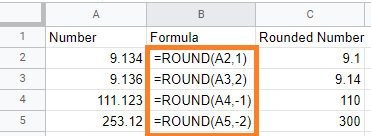In the above example,

• The decimal value (9.134, 1) is rounded to 9.1. Since we are specifying the places with “1” only one value is returned.
• If the second number is greater than 5 then the value is increased, i.e., = ROUND(9.165,1) gives you 9.2
• If the second number is lesser than 5, then the value is returned as the same, i.e., =ROUND(9.134,1) gives you 9.1
• The decimal value (9.136, 2) is rounded to 9.14. Since the third value is greater than 5, the second number is rounded up.
• The decimal value (111.123, -1) gives you 110. Here Google sheets round function will round up to 10s, 100s, and so on if you use negative place value. Since the 111.123 decimal number is round down to the number 110
• The decimal value (253.12,-2)  gives you 300. Since the nearest value to the (253.12, -2) number is 300. If we have the number (243.12, -2)  then the results would be 200

### ROUNDUP Function in Google Sheets

ROUNDUP function in google sheets helps you to round up the number as specified in places. This ROUNDUP function is similar to the ROUND function in google sheets.

Syntax:

=ROUNDUP(value, places)

• value – we should specify the decimal number which has to be rounded up
• places -we should specify the decimal place value that has to round up

Example:

• =ROUNDUP(2.623, 0) – This will give you 3
• =ROUNDUP(1.134, 1) – This will give you 1.2
• =ROUNDUP(3.906, 2) – This will give you 3.91
• =ROUNDUP(111.568, -1) – This will give you 120
• =ROUNDUP(253.555, -2) – This will give you 300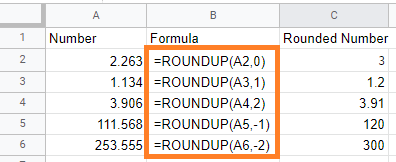In the above example,

• The decimal value (2.263, 0) is rounded to 3. Since the right side number is greater than 5, it is rounded to the next greater integer number.
• The decimal value(1.134, 1) is rounded up to 1.2. Here, the value of the place is mentioned as “1”. It returns the first right number on the right side.
• If the next number is greater than 5 then it will be rounded up
• If the next number is lesser than 5 then it will be rounded down
• The decimal value(3.906, 2) is rounded up to 3.91. Here, the right side of the third number is greater than 5 so the second number is rounded up.
• The decimal value (111.568, -1) is rounded up to 120. The negative value of the places will be rounded up to the nearest 10’s or 100’s.
• The decimal value of (253.555, -2) is rounded up to 300. Since the left side number is greater than 250 it is rounded up to 300. If it is less than 250 i.e., (241.555, -2) it is rounded up to 200.

ROUNDDOWN function in google sheets helps you to round down the number as specified in places. This ROUNDDOWN function is similar to the ROUND function in google sheets.

Syntax:

=ROUNDDOWN(value, places)

• value – we should specify the decimal number which has to be rounded down
• places -we should specify the decimal place value that has to round down

Example:

• =ROUNDDOWN(2.623, 0) – This will give you 2
• =ROUNDDOWN(1.134, 1) – This will give you 1.1
• =ROUNDUDOWN(3.906, 2) – This will give you 3.9
• =ROUNDDOWN(111.568, -1) – This will give you 110
• =ROUNDDOWN(253.555, -2) – This will give you 200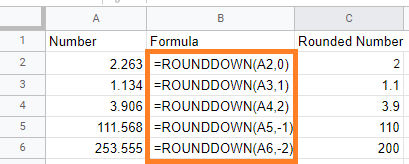In the above example,

• The decimal value (2.263, 0) is rounded down to 2. Even if the right side number is greater than 5, the ROUNDDOWN function in google sheets will round down.
• The decimal value(1.134, 1) is rounded down to 1.1. Here, the value of the place is mentioned as “1”. It returns the first right number on the right side.
• The decimal value(3.906, 2) is rounded down to 3.9. Here, the right side of the third number is 0, hence it is ignored.
• The decimal value (111.568, -1) is rounded down to 110. The negative value of the places will be rounded up to the nearest 10’s or 100’s.
• The decimal value of (253.555, -2) is rounded down to 200. Here, the nearest 100’s to the given number is 200.

### MROUND Function in Google Sheets

Using the multiple round functions in Google Sheets, you can take your rounding capabilities to the next level. Instead of a decimal position, these functions round to the nearest multiple of a given factor.

Syntax:

=MROUND(value,factor)

MROUND function in google sheets will round to the factor’s nearest multiple.

Example:

• =MROUND(14, 5) – This will give 15.
• =MROUND(12, 5) – This will give 10.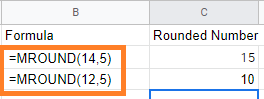In the above example,

The MROUND function in google sheets will round to the nearest multiple of 5.

• The number 14 is nearest to 15 in 5 multiple tables.
• The number 12 is nearest to 10 in 5 multiple tables.

### Round Number with the Formula in Google Sheets

Google Sheets allows you to return a rounded result by using ROUND as a wrapper.

For example, suppose we want to add the values in cells “A2” and “B2,” but we want the result rounded to the nearest whole number.

To accomplish this, we can use the formula “=SUM(A2:B2)” to calculate the value of “A2” plus “B2.” We can enclose that formula within =ROUND as follows:

=ROUND(SUM(4.44, 5.71) – This will give you 10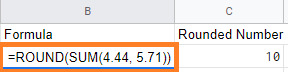Here, 4.44 is rounded to 4, and 5.71 is rounded to 6, hence we get the 10 as the result.

Google Sheets’ built-in rounding functions are an extremely versatile set of tools to use on your workbooks.